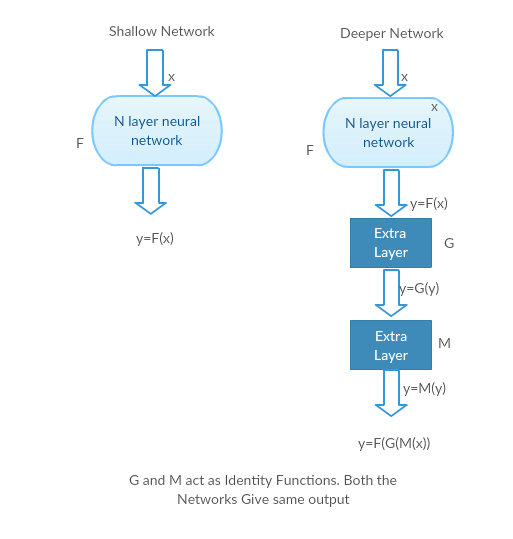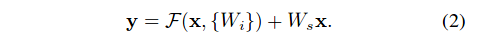# Understanding and Implementing Architectures of ResNet and ResNeXt for state-of-the-art Image Classification: From Microsoft to Facebook [Part 1]

## In this two part blog post we will explore Residual networks. More specifically we will discuss three papers released by Microsoft Research and Facebook AI research, state of the art Image classification networks- ResNet and ResNeXt Architectures and try to implement them on Pytorch.

This is Part 1 of two-part series explaining blog post exploring residual networks.

• Understanding and implementing ResNet Architecture [Part-1]
• Understanding and implementing ResNeXt Architecture[Part-2]

We will review the following three papers introducing and improving residual network:

## Was ResNet Successful?

• Won 1st place in the ILSVRC 2015 classification competition with top-5 error rate of 3.57% (An ensemble model)
• Won the 1st place in ILSVRC and COCO 2015 competition in ImageNet Detection, ImageNet localization, Coco detection and Coco segmentation.
• Replacing VGG-16 layers in Faster R-CNN with ResNet-101. They observed a relative improvements of 28%
• Efficiently trained networks with 100 layers and 1000 layers also.

# What problem does ResNet solve?

## Problem:

When deeper networks starts converging, a degradation problem has been exposed: with the network depth increasing, accuracy gets saturated and then degrades rapidly.

Let us take a shallow network and its deeper counterpart by adding more layers to it.

Worst case scenario: Deeper model’s early layers can be replaced with shallow network and the remaining layers can just act as an identity function (Input equal to output).Shallow network and its deeper variant both giving the same output

Rewarding scenario: In the deeper network the additional layers better approximates the mapping than it’s shallower counter part and reduces the error by a significant margin.

Experiment: In the worst case scenario, both the shallow network and deeper variant of it should give the same accuracy. In the rewarding scenario case, the deeper model should give better accuracy than it’s shallower counter part. But experiments with our present solvers reveal that deeper models doesn’t perform well. So using deeper networks is degrading the performance of the model. This papers tries to solve this problem using Deep Residual learning framework.

## How to solve?

Instead of learning a direct mapping of x ->y with a function H(x) (A few stacked non-linear layers). Let us define the residual function using F(x) = H(x) — x, which can be reframed into H(x) = F(x)+x, where F(x) and x represents the stacked non-linear layers and the identity function(input=output) respectively.

The author’s hypothesis is that it is easy to optimize the residual mapping function F(x) than to optimize the original, unreferenced mapping H(x).

## Intuition behind Residual blocks:

If the identity mapping is optimal, We can easily push the residuals to zero (F(x) = 0) than to fit an identity mapping (x, input=output) by a stack of non-linear layers. In simple language it is very easy to come up with a solution like F(x) =0 rather than F(x)=x using stack of non-linear cnn layers as function (Think about it). So, this function F(x) is what the authors called Residual function.

The authors made several tests to test their hypothesis. Lets look at each of them now.

## Test cases:

Take a plain network (VGG kind 18 layer network) (Network-1) and a deeper variant of it (34-layer, Network-2) and add Residual layers to the Network-2 (34 layer with residual connections, Network-3).

Designing the network:

1. Use 3*3 filters mostly.
2. Down sampling with CNN layers with stride 2.
3. Global average pooling layer and a 1000-way fully-connected layer with Softmax in the end.

There are two kinds of residual connections:

1. The identity shortcuts (x) can be directly used when the input and output are of the same dimensions.

2. When the dimensions change, A) The shortcut still performs identity mapping, with extra zero entries padded with the increased dimension. B) The projection shortcut is used to match the dimension (done by 1*1 conv) using the following formulaResidual block function when the input and output dimensions are not same.

The first case adds no extra parameters, the second one adds in the form of W_{s}

Results:

Even though the 18 layer network is just the subspace in 34 layer network, it still performs better. ResNet outperforms by a significant margin in case the network is deeper

## Deeper Studies

The following networks are studied

Each ResNet block is either 2 layer deep (Used in small networks like ResNet 18, 34) or 3 layer deep( ResNet 50, 101, 152).

Pytorch Implementation can be seen here:

The Bottleneck class implements a 3 layer block and Basicblock implements a 2 layer block. It also has implementations of all ResNet Architectures with pretrained weights trained on ImageNet.

## Observations:

1. ResNet Network Converges faster compared to plain counter part of it.
2. Identity vs Projection shorcuts. Very small incremental gains using projection shortcuts (Equation-2) in all the layers. So all ResNet blocks use only Identity shortcuts with Projections shortcuts used only when the dimensions changes.
3. ResNet-34 achieved a top-5 validation error of 5.71% better than BN-inception and VGG. ResNet-152 achieves a top-5 validation error of 4.49%. An ensemble of 6 models with different depths achieves a top-5 validation error of 3.57%. Winning the 1st place in ILSVRC-2015